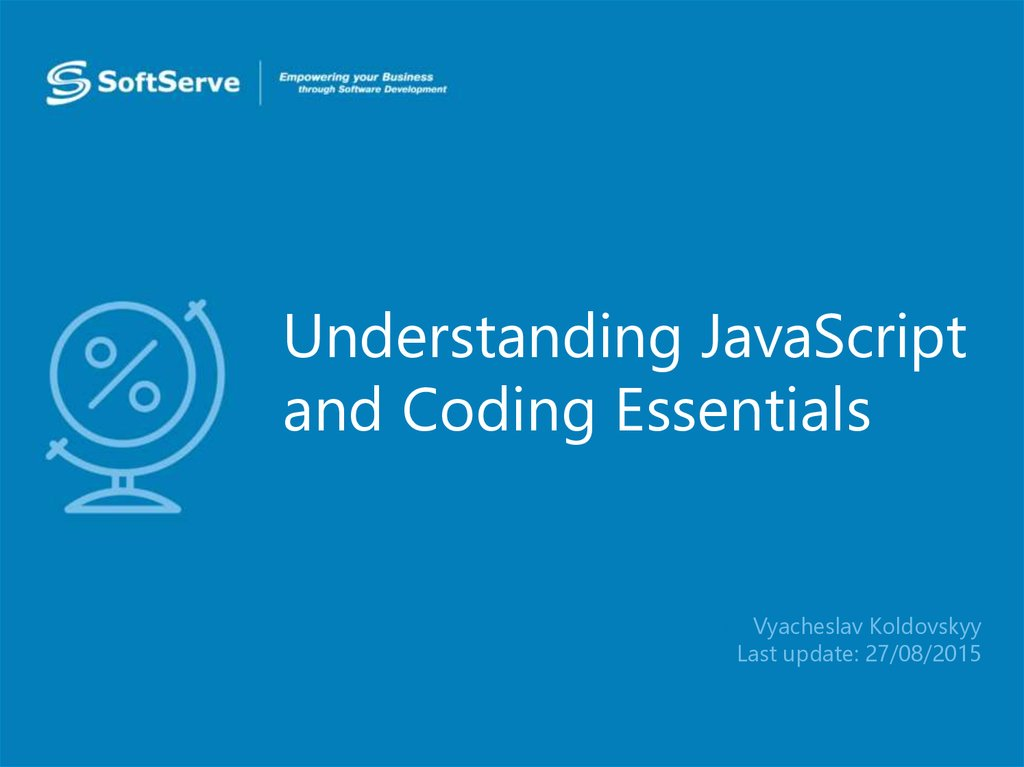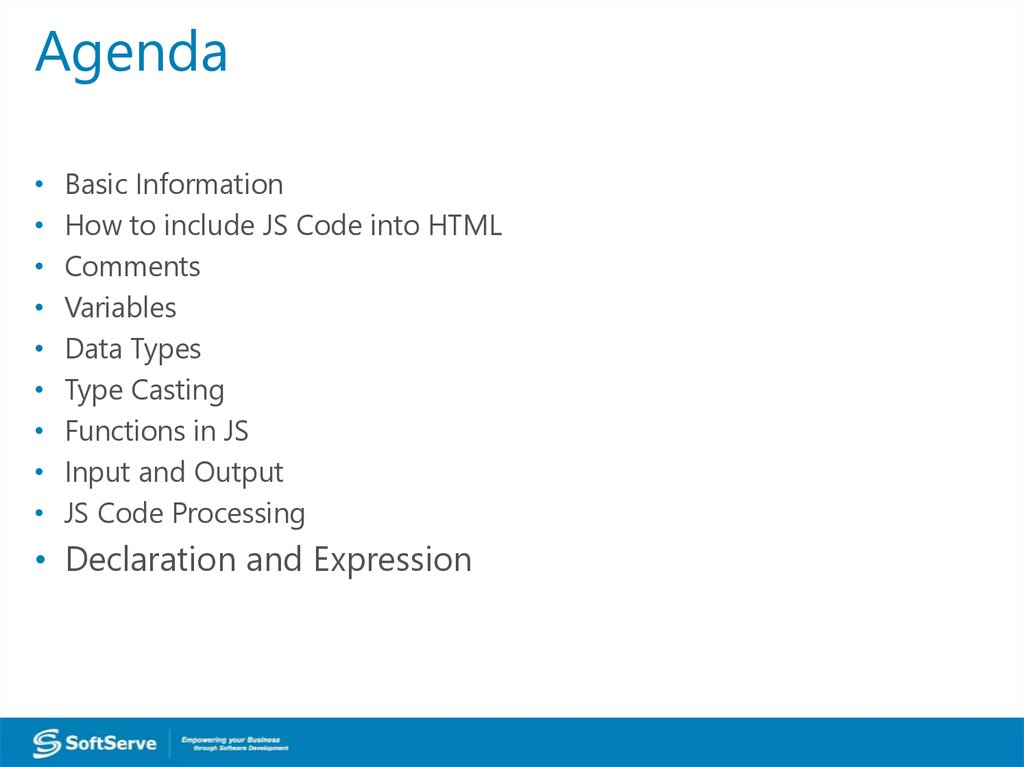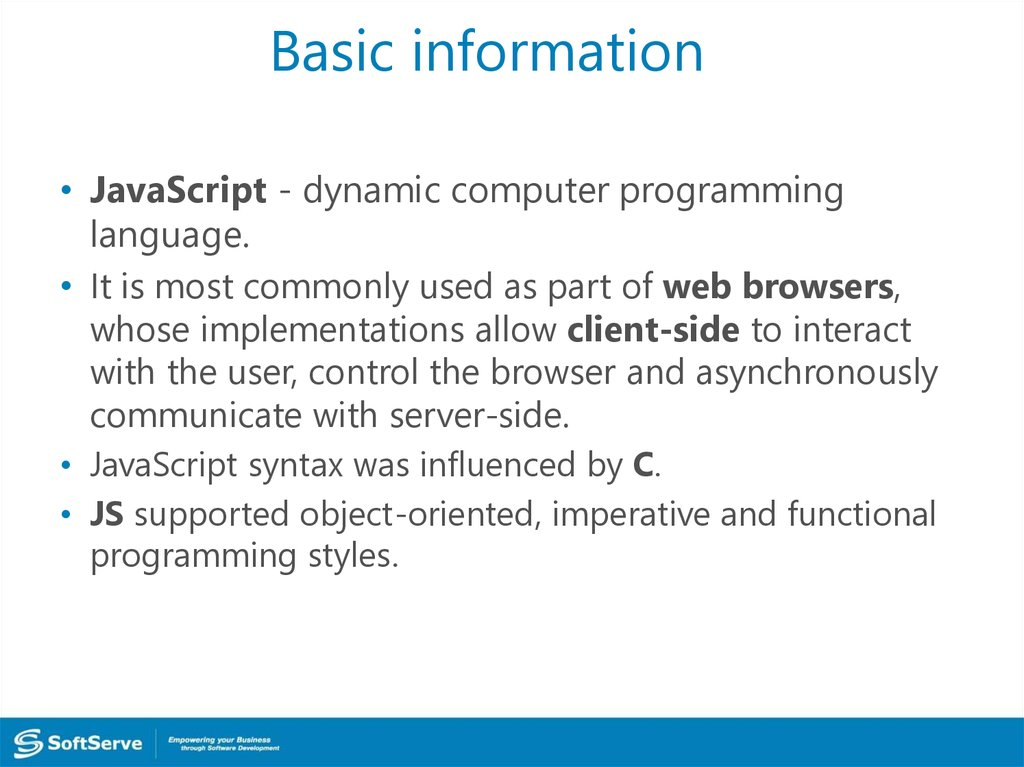# Understanding JavaScript and Coding Essentials

## 1. Understanding JavaScript and Coding Essentials

• Vyacheslav Koldovskyy
Last update: 27/08/2015

## 2. Agenda

Basic Information
How to include JS Code into HTML
Variables
Data Types
Type Casting
Functions in JS
Input and Output
JS Code Processing
• Declaration and Expression

## 3. Basic information

• JavaScript - dynamic computer programming
language.
• It is most commonly used as part of web browsers,
whose implementations allow client-side to interact
with the user, control the browser and asynchronously
communicate with server-side.
• JavaScript syntax was influenced by C.
• JS supported object-oriented, imperative and functional
programming styles.

## 4. How to add JavaScript to HTML?

1. Inline JavaScript:
2. Internal tag <script>:
<script>
</script>
3. External file:
<script src="script.js"></script>

Comments - part of the program text which will be
ignored by language interpreter 
The /* characters, followed by any sequence of
characters (including new lines), followed by
the */ characters. 
The // characters, followed by any sequence of
characters, but only in current line. Therefore, it is
commonly called a "single-line comment." 

## 6. Variables

Variable – symbolic name associated with a value
and whose associated value may be changed. 
Declaration – process of variable's specifying.
Usually declaration consist of defining: type, name
and default value of variable. 
A process in which a variable is set to its first value is
called initialization. 

## 7. Declaration and initialization

var – special keyword for declaration of variables
In JavaScript
var variable;
variable = 10;
//declaration
//initialization

Or quickly
var variable = 10;



## 8. Global and local

JavaScript has two types of variables:
global - exist in memory and is available at all times
of the program. In JS it's a variables of page. 
local - exist in memory and is available only in block
when variable is defined. In JS it's defined in function
variables. 

## 9. Data types

JavaScript has 6 base data types:
• Number – scalar type for integer and real digits
• Boolean – scalar type for logical values
• String – special type for work with text information
• Undefined – special type for uninitialized variables
• Null – special type for "cleaning" of variables
• Object – complex type for service and user needs

## 10. Number, Boolean and String

var n = 10; or var n = Number(10);
//number values for example: -1, 10, 3.14, Nan, Infinity
var b = true; or var b = Boolean(true);
//bollean values: true and false
var s = “text”; or var s = String(“text”);
//string values for example: “”, “text”, ‘text’




## 11. Null and Undefined

var n = null;
//null variables can have only null value

var u;
// created and uninitialized
And Object type… but it will be reviewed in future :)

## 12. Type casting

There are two types of casting:
var a, b, c;
a = 10;
b = true;
c = a + b;

Explicit
Implicit

var a, b, c;
a = 10;
b = true;
c = a + Number(b);
But both ways given c =11 as a result!


## 13. Type casting

Rules of typing casting:




All scalar types try to convert itself to largest scalar
type: Boolean to Number, Number to String.
If Boolean converted to String it at first converted to
Number and after them Number to String.
In mathematical operations (excluding +) String
should be converted to Number.
Null and Undefined converted to String as “null” and
“undefined”, and to Number as a 0 and NaN

## 14. Functions

In mathematics:
Function is a relation between a set of inputs and a set

of permissible outputs.
y = f(x)

In classical programming
Function is a named part of a code that performs a

distinct service.

## 15. Example

var i, base, power, result;

base = 2; power = 2; result = 1; 
for(i = 0; i < power; i++) { 
result *= base;
}
console.log(result); 
base = 3; power = 4; result = 1;
for(i = 0; i < power; i++) {
result *= base;
}
console.log(result);


## 16. Function Declaration

function name (a, b) {
return a + b;
}

* you can return one value only

* return always interrupts the execution.

* place your return at the end of a function


## 17. Function call

Call - operation for execution of function.
( ) – operator for this action.


Usually function can be called by name.


## 18. Example

var out;
out = pow(2, 2);
console.log(out);
out = pow(3, 4);
console.log(out);
function pow (base, power) {
var result = 1;
for(var i = 0; i < power; i++) {
result *= base;
}
return result;
}

## 19. Code processing

var a = 10;
test();
function test () {
a = 30;
var b = 40;
}
var b = 20;
console.log(a, b);

## 20. Code processing

1.
var a = 10;
test();
function test () {
a = 30;
var b = 40;
}
var b = 20;
console.log(a, b);

## 21. Code processing

2.
1.
3.
var a = 10;
test();
function test () {
a = 30;
var b = 40;
}
var b = 20;
console.log(a, b);

## 22. Code processing

2.
4.
1.
5.
3.
6.
var a = 10;
test();
function test () {
a = 30;
var b = 40;
}
var b = 20;
console.log(a, b);

## 23. Code processing

2.
4.
1.
5.
3.
6.
var a = 10;
test();
function test () {
5.2
a = 30;
var b = 40; 5.1
}
var b = 20;
console.log(a, b);

## 24. Function Declaration and Expression

function name () {
body;
}
var name = function () {
body;
};



Functions in JavaScript are Objects.
As a result, functions are accessible by
reference.


Functions can be used as a parameter in other

function.
References to functions can be saved in any
other variable.

## 26. Program flow

Operators in a program processed in linear order:
from top to bottom and from left to right.

Such sequence is called Program flow.
There are several methods intended to change
JavaScript has conditions, loops and switch statement.


## 27. Conditions: if-else

Very often we have to choose Most of algorithms have
situation when next step related of some conditions depended
 statement.
on previous steps. It's a reason to use if-else
if (condition) {

true branch;
} else {
false branch;
}
if (condition) {
true branch;
}


## 28. Conditions: if-else

Example:
Function get a parameter
discount. And if discount is
"silver" or "gold“, function
modifies global variable
price.
In
this
example
a
shortened form of operator
was used.
function discount (type) {
if (type === “silver”) {
price *= 0.9;
}
if (type === “gold”) {
price *= 0.85;
}
return price;
}

## 29. Conditions: ?:

Sometimes if-else too bulky. If we need to initialize a
variable modifying it by simple conditions; or we
need to return a value from function and this value is
dependent on something, we can use ternary
Ternary operator like ?:.
result = (condition)? true action: false action;
Let’s rewrite the last example using ternary operator.


## 30. Conditions: ?:

We get a more compact
function discount (type) {
if (type === “silver”) {
So be careful!
price *= 0.9;
}
if (type === “gold”) {
price *= 0.85;
}
function discount (type) {
return price;
price *= (type === “silver”)? 0.9: 1;
}
price *= (type === “gold”)? 0.85: 1;
}
return price;

## 31. Loops: for

Loops are used when algorithm requires repeating of
statements.

First of them: for - loop with counter
for (start position; repeat condition; step) {
body of loop; // will be repeated 
}
One processing of loop’s body is called iteration. 

## 32. Loops: while and do-while

Two others types of loops: while and do-while

while (condition) {
body of loop;
}
do {
body of loop;
} while (condition);
The main difference between these loops is the moment of
condition calculation. While calculates condition, and if the
result is true, while does iteration. Do-while initially does

iteration and after that calculates
a condition.

## 33. Loops: examples

Example 1:
Text with number
of current iteration
will be print 5
times
for (var i = 0; i < 5; i++) {
console.log(“Iteration # %d”, i + 1);

}
Example 2:
This loop will be
repeated
until
accumulation
reaches 100 or gets
grater value.
while (accumulation < 100) {
accumulation += doSomething();

}

## 34. Loops: break and continue

There are two keywords for loops control :
• break – aborts loop and moves control to next
statement after the loop;
• continue – aborts current iteration and
immediately starts next iteration.
Try not to use this keywords. A good loop have one
entering point, one condition and one exit.

## 35. Switch

Switch statement allows to select one of many blocks of code
to be executed. If all options don’t fit, default statements will
be processed
switch (statement) {
case value1: some body;
break;
case value2: some body;
break;
...
default: some body;
}

## 36. Switch

Example:
This switch looks
for
the
word
equivalent for a
mark in the 5point system
Default statement
is not used.
switch (mark) {
case 5: result = “excellent”;
break;
case 4: result = “good”;
break;
case 3: result = “satisfactorily”;
break;
break;
}